Home > CC2 > Chapter 7 > Lesson 7.2.2 > Problem7-115

7-115.
1. For each equation below, solve for x. Sometimes the easiest strategy is to use mental math. Homework Help ✎

1. x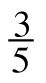= 1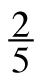2. 5.2 + x = 10.95

3. 2x − 3.25 = 7.15

4.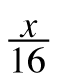=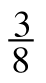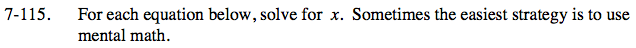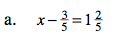$\text{What does }\frac{3}{5}+\frac{2}{5}=?$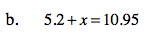x = 5.75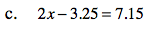x = 5.2
Be sure to know how to get this answer using mental math. Recognize that 3.25 + 7.15 equals an even decimal, 10.4.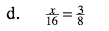8(2) = 16
What must x be?